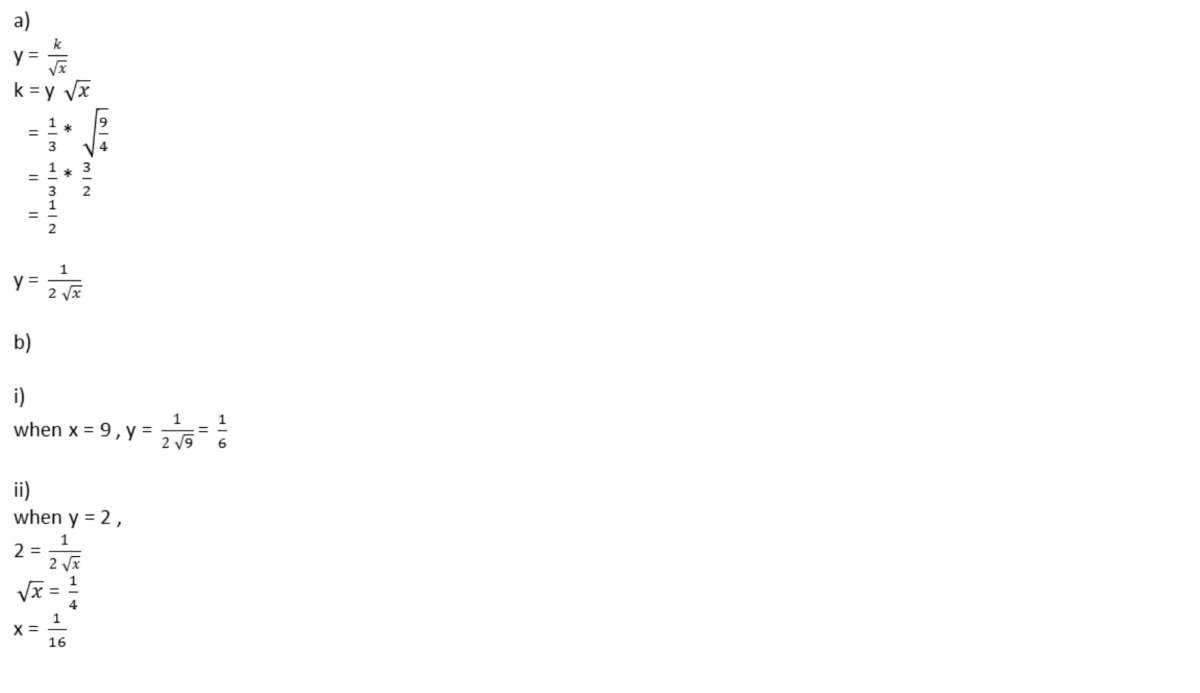# Question

Ps help. Many tks and God Bless:)

Given that y is inversely proportional to the square root of x, and y =1/3 when x = 2 1/4,

(a) find the relation between x and y,

(b) calculate the value of

(i) y when x=9

(ii) x when y =2